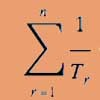Search by Topic

Resources tagged with Triangle numbers similar to Seriesly:

Filter by: Content type:
Age range:
Challenge level:

There are 12 results

Broad Topics > Numbers and the Number System > Triangle numbersSpeedy Summations

Age 16 to 18 Challenge Level:

Watch the video to see how to add together an arithmetic sequence of numbers efficiently.Reciprocal Triangles

Age 16 to 18 Challenge Level:

Prove that the sum of the reciprocals of the first n triangular numbers gets closer and closer to 2 as n grows.Slick Summing

Age 14 to 16 Challenge Level:

Watch the video to see how Charlie works out the sum. Can you adapt his method?Iff

Age 14 to 18 Challenge Level:Take Ten Sticks

Age 11 to 16 Challenge Level:

Take ten sticks in heaps any way you like. Make a new heap using one from each of the heaps. By repeating that process could the arrangement 7 - 1 - 1 - 1 ever turn up, except by starting with it?Triangles Within Triangles

Age 14 to 16 Challenge Level:

Can you find a rule which connects consecutive triangular numbers?Triangles Within Squares

Age 14 to 16 Challenge Level:

Can you find a rule which relates triangular numbers to square numbers?Mystic Rose

Age 14 to 16 Challenge Level:

Use the animation to help you work out how many lines are needed to draw mystic roses of different sizes.Series Sums

Age 14 to 16 Challenge Level:

Let S1 = 1 , S2 = 2 + 3, S3 = 4 + 5 + 6 ,........ Calculate S17.Triangular Triples

Age 14 to 16 Challenge Level:

Show that 8778, 10296 and 13530 are three triangular numbers and that they form a Pythagorean triple.Triangles Within Pentagons

Age 14 to 16 Challenge Level:

Show that all pentagonal numbers are one third of a triangular number.Reasonable Algebra

Age 14 to 16 Challenge Level:

Use algebra to reason why 16 and 32 are impossible to create as the sum of consecutive numbers.